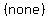# #84 5.05 Precalculus questionsAnonymous
timer Asked: May 15th, 2015
account_balance_wallet \$5

### Question Descriptionvicky david
School: New York University```                                            ?
cos4x - sin4x = cos(2x)
Factor the left
side as the
difference of
two squares
?
(cos²x - sin²x)(cos²x + cos²x) = cos(2x)

Use the identity
cos²q + sin²q = 1
to replace the second
parentheses on left
by 1.
?
(cos²x - sin²x)(1) = cos(2x)

?
cos²x - sin²x = cos(2x)
Use the identity
cos(2q) = cos²q - sin²q
to replace the left
side.
Ö
cos(2x) = cos(2x)
------------------------------------------------------------

For all the others you first have to know that

sin-1s means "the angle with the smallest absolute value
whose sine is s"

cos-1c means "the angle with the smallest absolute value
whose cosine is c"

tan-1t means "the angle with the smallest absolute value
whose tangent is t"

And in cases where there are two angles which are the
smallest in absolute value, one positive and one negative,
we always choose the positive angle.

-----------------------------------------

How do you find cos(sin-1())

First we look only at what is in the parentheses.
We see this: sin-1(). This means an angle
whose sine is.  The sine is negative in
quadrants III and IV.  An angle in quadrant IV
will have a smaller absolute value if rotated
clockwise as a negative angle than a positive
angle in quadrant III, so sin-1() is an
angle in quadrant IV, so we draw a radius vector
in quadrant IV to represent the angle sin-1()Next we draw a perpendicular from the end of that
radius vector directly to the x-axis, like this:Next we indicate the horizontal side of the
resulting right triangle as x, the vertical side as
y, and the radius vector or hypotenuse as r.Now that angle's sine is.  We know that the
sine of an angle isor,
so we select the numerator of the fractionto be
y or the opposite, and the denominator of the fractionto be r or the hypotenuse, which is the radius vector.  Since
the r or hypotenuse is ALWAYS POSITIVE, we must make the y-value
negative, which is correct because y goes downward below the
x-axis.  So we have y = -5 and r = 13.  We label these:Now we must find x by the Pythagorean theorem

x² + y² = r²
x² + (-5)² = (13)²
x² + 25 = 169
x² = 169 - 25
x² = 144
___
x = ±Ö144
x = ±12

and since x goes to the right we use the
positive value and x = 12. So we label
x as 12:Now go back to the original expression:

cos(sin-1())

We have drawn the angle sin-1() and
found the values of x the adjacent, y the opposite,
and r the hypotenuse, and since,

cos(sin-1()) ==```

flag Report DMCAReviewAnonymous
awesome work thanksBrown University

1271 TutorsCalifornia Institute of Technology

2131 TutorsCarnegie Mellon University

982 TutorsColumbia University

1256 TutorsDartmouth University

2113 TutorsEmory University

2279 TutorsHarvard University

599 TutorsMassachusetts Institute of Technology

2319 TutorsNew York University

1645 TutorsNotre Dam University

1911 TutorsOklahoma University

2122 TutorsPennsylvania State University

932 TutorsPrinceton University

1211 TutorsStanford University

983 TutorsUniversity of California

1282 TutorsOxford University

123 TutorsYale University

2325 Tutors